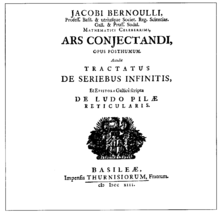Abstract: 本文介绍《推测术》前三部分，主要是古典概率的系统化和深化
Keywords: 推测术，猜度术，排列，古典概率，全概率公式

# 《推测术》前三部分内容提要《推测术》全书239页，分四部分：

“大数定律”在本书中没有得到命名，而是 1837年 泊松的一篇著作中提到了“大数定律”这个名词。

《推测术》前三部分，是古典概率的系统化和深化，比前面概率论的进步在于脱离了赌博等具体问题，而是着重与计算的一般规律和数学证明。完全和现代教材一致，并且其中意识到一些关键条件，比如，在重复扔骰子的描述中，书中表示，每次扔骰子获得点数的概率是不变的，这在以前被默认而无人特别说明，而伯努利指出了这一点，所以复合这种条件的模型被称为伯努利模型。他指明了独立概率下的乘法定理的表达方式，并在此基础上严格的证明了二项概率公式。

$$\sum^{n}_{i=1}i^{m}=\frac{n^{m+1}}{m+1}+\frac{n^m}{2}+\sum^{\frac{m}{2}}_{i=1}\frac{1}{2i}B_{2i}C^{m}_{2i-1}n^{m-2i+1}$$

$$B_2=\frac{1}{6},B_4=-\frac{1}{30},B_6=\frac{1}{42},B_6=-\frac{1}{30}$$

$$\frac{1}{2}=\frac{1}{2k+1}+\sum^{k}_{i=1}\frac{1}{2i}B_{2i}C^{2k}_{2i-1},k=1,2,\dots$$

A,B 两个人打网球，每局A胜的概率为 $p$ 对应的 B 获胜的概率 $q$ ，并且满足 $p>0,q>0,p+q=1$ 规定：当一方领先不少于2局，且领先一方至少胜4局时，该方取胜，求A取胜的概率。

$$h(i,j)=ph(i+1,j)+qh(i,j+1)\\ \text{set: }r=\frac{p}{q}=\frac{p}{1-p}$$

$$h(3,3)=p^2h(5,3)+2pqh(4,4)+q^2h(3,5)\\ =p^2+2pqh(3,3)$$

$$h(3,3)=\frac{p^2}{p^2+q^2}=\frac{r^2}{r^2+1}$$

$$h(2,3)=ph(3,3)+qh(2,4)=\frac{pr^2}{r^2+1}$$

$$\frac{r^7+5r^6+11r^5+15r^4}{r^7+5r^6+11r^5+15r^4+15r^3+11r^2+5r+1}$$

0%